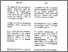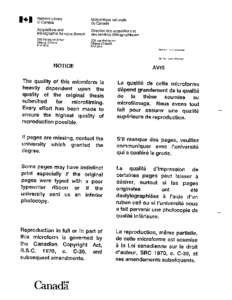Title:

# End depth and flow relationships for smooth open trapezoidal and circular channels

Ahlen, Karl (1994) End depth and flow relationships for smooth open trapezoidal and circular channels. Masters thesis, Concordia University.Preview
Text (application/pdf)
MM90814.pdf
2MB

## Abstract

The current study's objective was to obtain an accurate relationship between the end depth Y$\sb{\rm e}$ and the discharge rate for smooth open channels with trapezoidal and circular cross sections. To accomplish this, the momentum equation was used. The velocity and pressure head distributions at the end depth Y$\sb{\rm e}$ were determined to obtain the corresponding velocity and pressure coefficients, (alpha $\alpha$, beta $\beta$ and K). The pressure head readings were obtained through the use of a static Pitot tube. Velocity readings were obtained through the use of a Pitot tube and a Laser Doppler Anemometer (LDA) system. The velocity measurements with the Pitot tube utilised procedures to take into account the curvilinear nature of the flow in the Y$\sb{\rm e}$ region. The present investigation improves on existing studies in several ways. (1) The velocities over the entire end depth and critical depth sections for circular and trapezoidal cross sectional channels were measured over a range of flow conditions. (2) The pressure distribution at the end depth section for circular and trapezoidal cross sectional channels were measured to incorporate the pressure force term in the momentum analysis. (3) The pressure and velocity coefficients K, $\alpha$, and $\beta$ were evaluated based on the velocity and pressure distribution data. (4) Pressure head values were also calculated indirectly at the end depth section using velocity distribution data for circular and trapezoidal cross sectional channels. Another aspect of the study was the improved analysis of the momentum equation with the calculation of $\alpha$ and $\beta$ coefficients for both circular and trapezoidal channels. The improved analysis of the momentum equation provides for a more accurate functional relationship between the two flow variables. This would permit the establishment of a simple method for monitoring the flow rate in existing channels at their discharge point.

Divisions: Concordia University > Gina Cody School of Engineering and Computer Science > Building, Civil and Environmental Engineering Thesis (Masters) Ahlen, Karl xiii, 119 leaves : ill. ; 29 cm. Concordia University M.A. Sc. Dept. of Civil Engineering. Concordia University 1994 Balachandar, R TC 175 A35 1994 62 Concordia University Library 27 Aug 2009 17:09 13 Jul 2020 19:45 https://concordiauniversity.on.worldcat....
All items in Spectrum are protected by copyright, with all rights reserved. The use of items is governed by Spectrum's terms of access.

Repository Staff Only: item control pageResearch related to the current document (at the CORE website)
Back to top